Courses

# Test: Balancing of Single & Multi Cylinder Engines & Linear Vibration Analysis of Mechanical Systems - 1

## 20 Questions MCQ Test RRB JE for Mechanical Engineering | Test: Balancing of Single & Multi Cylinder Engines & Linear Vibration Analysis of Mechanical Systems - 1

Description
This mock test of Test: Balancing of Single & Multi Cylinder Engines & Linear Vibration Analysis of Mechanical Systems - 1 for Mechanical Engineering helps you for every Mechanical Engineering entrance exam. This contains 20 Multiple Choice Questions for Mechanical Engineering Test: Balancing of Single & Multi Cylinder Engines & Linear Vibration Analysis of Mechanical Systems - 1 (mcq) to study with solutions a complete question bank. The solved questions answers in this Test: Balancing of Single & Multi Cylinder Engines & Linear Vibration Analysis of Mechanical Systems - 1 quiz give you a good mix of easy questions and tough questions. Mechanical Engineering students definitely take this Test: Balancing of Single & Multi Cylinder Engines & Linear Vibration Analysis of Mechanical Systems - 1 exercise for a better result in the exam. You can find other Test: Balancing of Single & Multi Cylinder Engines & Linear Vibration Analysis of Mechanical Systems - 1 extra questions, long questions & short questions for Mechanical Engineering on EduRev as well by searching above.
QUESTION: 1

### Consider the triangle formed by the connecting rod and the crank of an IC engine as the two sides of the triangle. If the maximum area of this triangle occurs when the crank angle is 75°, the ratio of connecting rod length to crank radius is

Solution: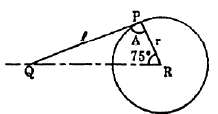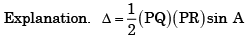Area will be max imum when A = 90°
i.e. PQR is a righ tan gled traingle.
∴ Ratio of connecting rot length to crank radius,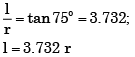QUESTION: 2

### A simple pendulum of length 5 m, with a bob of mass 1 kg, is in simple harmonic motion as it passes through its mean position, the bob has a speed of 5 m/s. The net force on the bob at the mean position is [GATE-2005]

Solution:

Force at mean position is zero.

QUESTION: 3

### Assertion (A): The supply of fuel is automatically regulated by governor according to the engine speed.   Reason (R): The automatic function is the application of d' Alembert's principle.

Solution:
QUESTION: 4

The natural frequency of a spring-mass system on earth is ωn .The natural frequency of this system on the moon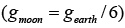Solution: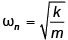neither mass nor stiffness depends on gravity. If you think about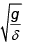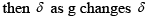will also change by same factor.

QUESTION: 5

The Klein's method of construction for reciprocating engine mechanism.

Solution:

Klein's method of construction for reciprocating engine mechanism is based on the acceleration diagram.

QUESTION: 6

The differential equation governing the vibrating system is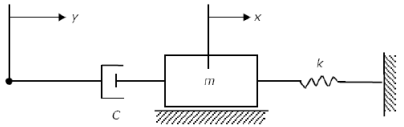Solution:

This is the differential equation governing the above vibrating system.

QUESTION: 7

The given figure shows the Klein's construction for acceleration of the slider-crank mechanism Which one of the following quadrilaterals represents the required acceleration diagram?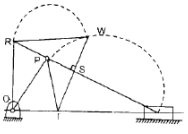Solution:
QUESTION: 8

A mass m attached to a light spring oscillates with a period of 2 sec. If the mass is increased by 2 kg, the period increases by 1sec. The value of m is

Solution: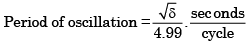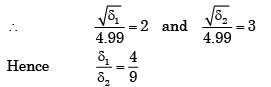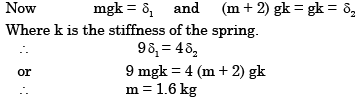QUESTION: 9

Figure shows Klein's construction for slider-crank mechanism OCP drawn to full scale. What velocity does CD represent?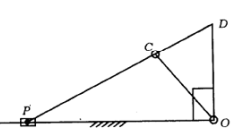Solution:

Velocity of crank pin (Vc) = OC
Velocity of piston (Vp) = OD
Velocity of piston with respect  to crank pin (Vpc) = CD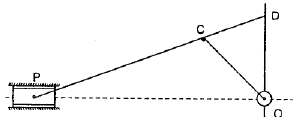QUESTION: 10

The above figure shows the schematic diagram of an IC engine producing a torque T = 40 N-m at the given instant. The Coulomb friction coefficient between the cylinder and the piston is 0.08. If the mass of the piston is 0.5 kg and the crank radius is 0.1 m, the Coulomb friction force occurring at the piston cylinder interface is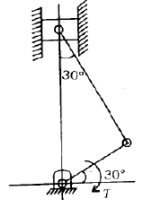Solution: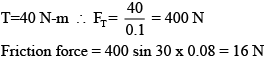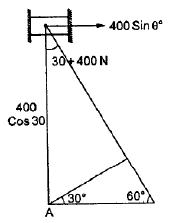QUESTION: 11

The natural frequency of the spring mass system shown in the figure is closest to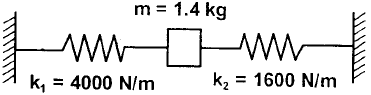Solution: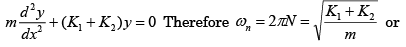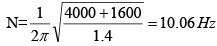QUESTION: 12

Klein's construction for determining the acceleration of piston P is shown in the given figure. When N coincides with K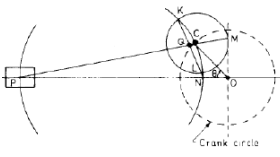Solution:
QUESTION: 13

Consider the arrangement shown in the figure below where J is the combined polar mass moment of inertia of the disc and the shafts. K1, K2, K3 are the torsional stiffness of the respective shafts. The natural frequency of torsional oscillation of the disc is given by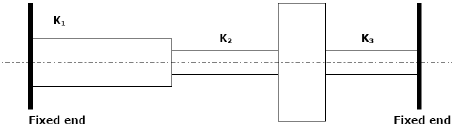Solution: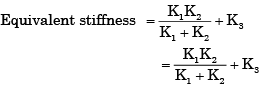QUESTION: 14

In a slider-crank mechanism the maximum acceleration of slider is obtained when the crank is

Solution:
QUESTION: 15

A uniform rigid rod of mass m = 1 kg and length L = 1 m is hinged at its centre and laterally supported at one end by a spring of constant k = 300 N/m. The natural frequency (ωn in rad/s is

Solution: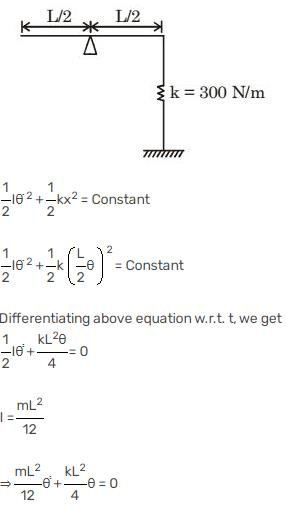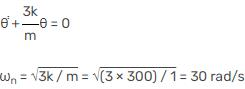QUESTION: 16

In a reciprocating engine mechanism, the crank and connecting rod of same length r meters are at right angles to each other at a given instant, when the crank makes an angle of 45° with IDC. If the crank rotates with a uniform velocity of ω rad/s, the angular acceleration of the connecting rod will be

Solution:

Angular acceleration of connecting rod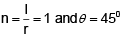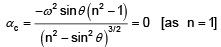QUESTION: 17

As shown in Figure, a mass of 100 kg is held between two springs. The natural frequency of vibration of the system, in cycles/s, is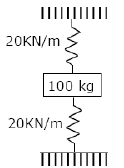Solution: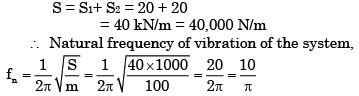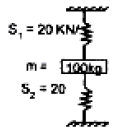QUESTION: 18

In a slider-bar mechanism, when does the connecting rod have zero angular velocity?

Solution: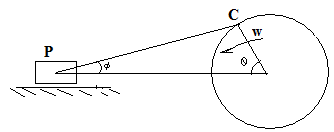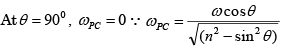QUESTION: 19

In the figure shown, the spring deflects by δ to position A (the equilibrium position) when a mass m is kept on it. During free vibration, the mass is at position B at some instant. The change in potential energy of the springmass system from position A to position B is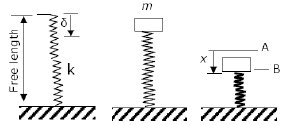Solution: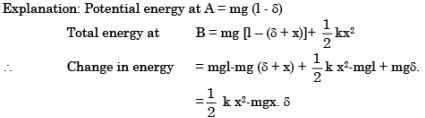QUESTION: 20

Which one of the following statements in the context of balancing in engines is correct?

Solution: